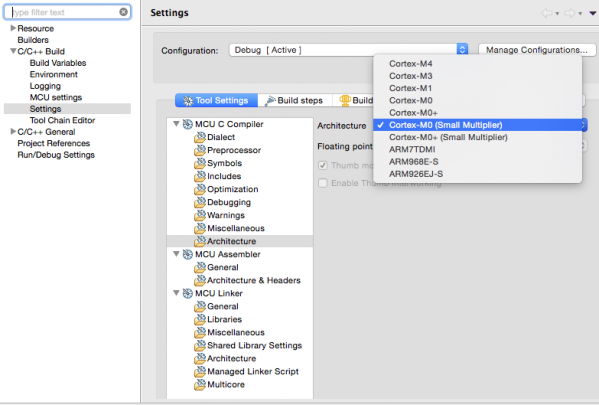# Use of Cortex-M0/M0+ multiply instructions on LPC43xx and LPC5410x

cancel
Showing results for
Show  only  | Search instead for
Did you mean:

## Use of Cortex-M0/M0+ multiply instructions on LPC43xx and LPC5410x

4,268 ViewsSenior Contributor I

## Multiplier Implementation

The Cortex-M0 and Cortex-M0+ CPU cores can be implemented with one of two hardware multiply options:

• Fast : This allows the MULS instruction to execute in a single cycle

• Small : An iterative multiplier that takes 32 cycles to execute a MULS instruction.

For most NXP MCU's which use these cores, the 'Fast' option is implemented. However the Cortex-M0 on LPC43xx and the Cortex-M0+ on LPC5410x implement the 'Small' option.

## Changing compiler behavior for 'Slow' multiplier implementation

To multiply two integer variables the GCC compiler used by LPCXpresso IDE will always use a MULS instruction.

But for a multiply by a constant, the compiler can either use a sequence of add / subtract / shift operations, or the MULS instruction.

By default, the compiler assumes that a 'Fast' multiplier option is implemented by the target hardware, thus it will use a MULS instruction as this is assumed to be fastest and smallest. However this is not actually the case for Cortex-M0 on LPC43xx and the Cortex-M0+ on LPC5410x, and in some cases it may be preferable to generate sequence of add / subtract / shift operations in order to obtain better performance.

In order to allow this, LPCXpresso 7.6 introduced a new mechanism to allow the user to instruct GCC to generate add / subtract / shift operations.

To turn this on, go to

Project -> Properties -> C/C++ Build -> Settings -> Tool Settings …
… -> MCU C Compiler -> Architecture

and select the small multiplier version of Cortex-M0:For example if this is done for the following simple function.

int mult (int a) {

return a * 42;

}

it will change the generated code (compiled -O1) from:

00000000 <mult>:

0:232a     movs   r3, #42; 0x2a

2:4358     muls   r0, r3

4:4770     bx     lr

6:46c0     nop    ; (mov r8, r8)

to:

00000000 <mult>:

0:0043     lsls   r3, r0, #1

4:00c3     lsls   r3, r0, #3

6:1a18     subs   r0, r3, r0

8:0040     lsls   r0, r0, #1

a:4770     bx     lr

## Notes

1. When deciding whether to change a MULS into multiple add/subtract/shift instructions, the compiler will not carry out the change if it would mean ending up with more than 5 instructions.
2. MULS instructions will not be changed into multiple add/subtract/shift instructions when compiing -Os, as when this option is used code size is considered to be more important than performance.
Labels (1)
• ### C C++ Library

Tags (4)
0 Replies